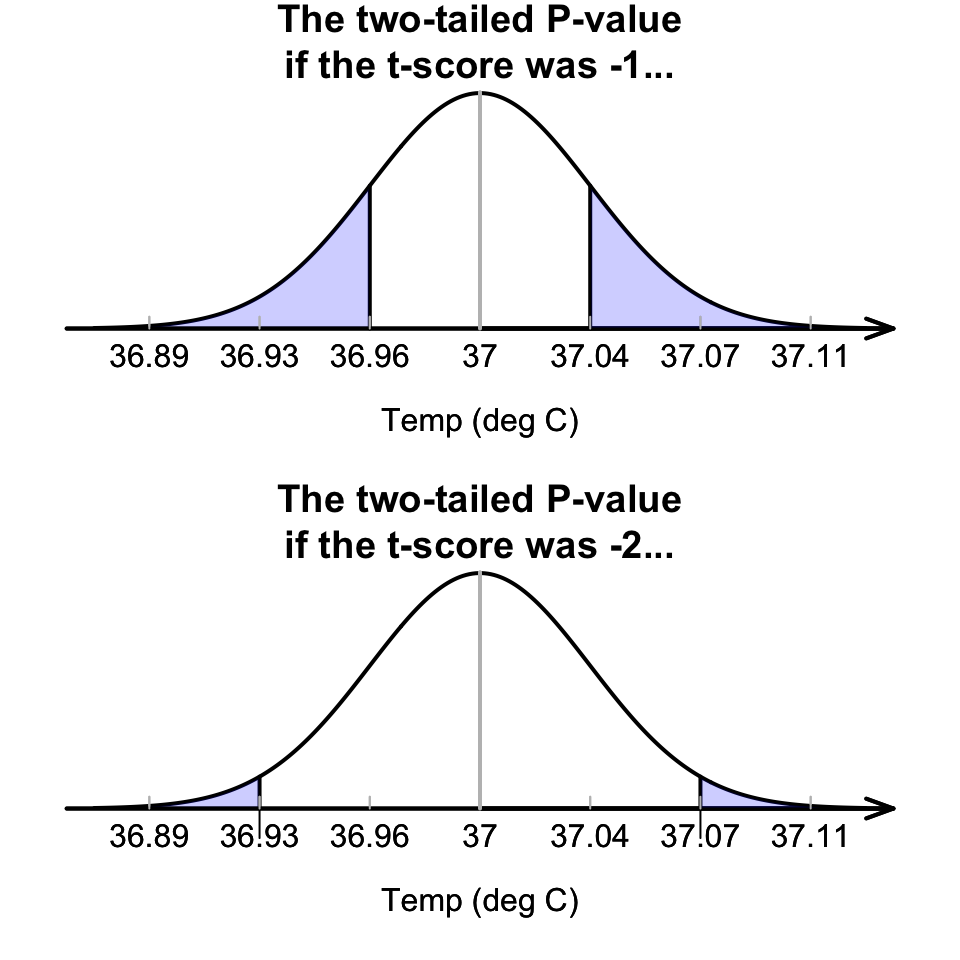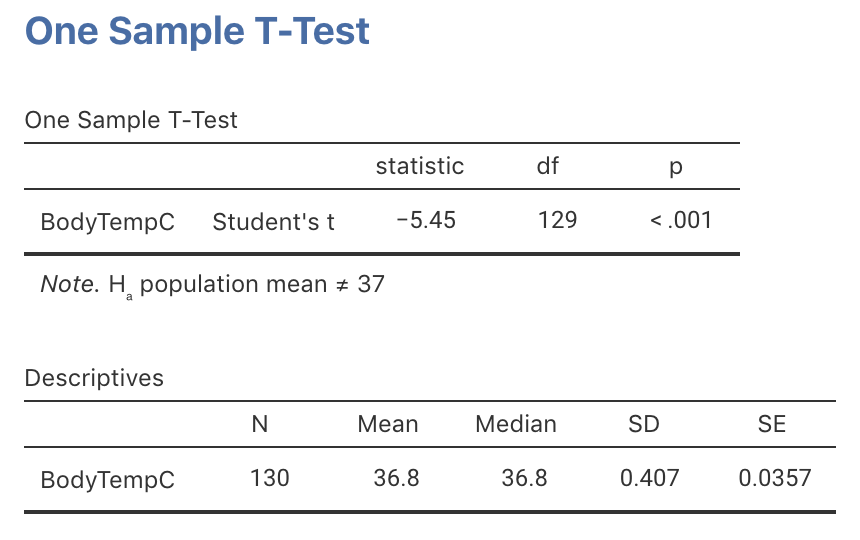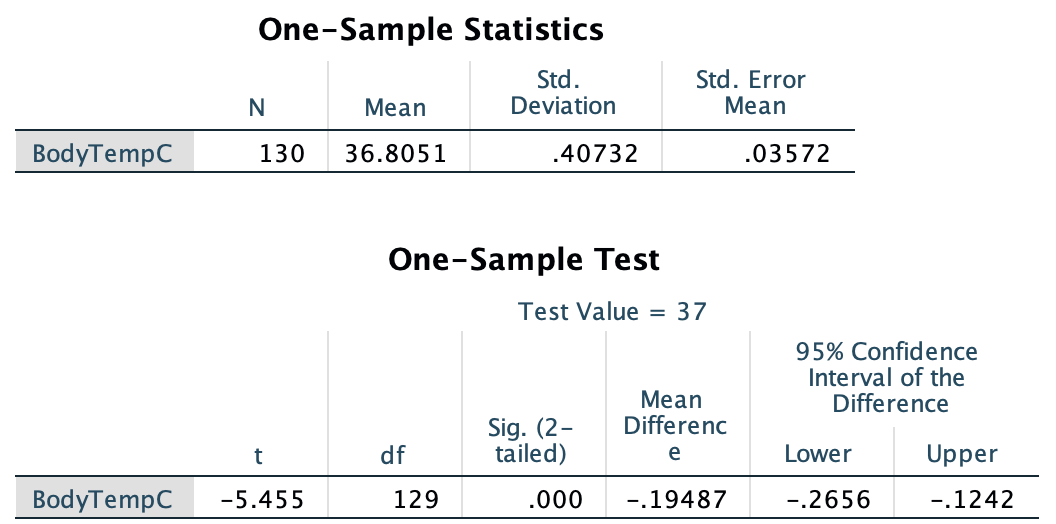## 27.5$$P$$-values: One mean

This is the decision-making progress so far:

1. Assume that the population mean is $$37.0^\circ\text{C}$$ (this is $$H_0$$).
2. Based on this assumption, describe what to expect from the sample means (Fig. 27.2).
3. The observed statistic is computed, relative to what is expected using a $$t$$-score (Fig. 27.3): $$t=-5.453$$.

The value of the $$t$$-score shows that the value of $$\bar{x}$$ is highly unusual. How unusual can be assessed more precisely using a $$P$$-value, which is used widely in scientific research. The $$P$$-value is a way of measuring how unusual an observation is (if $$H_0$$ is true).

$$P$$ values can be approximated using the 68–95–99.7 rule and a diagram (Sect. 27.5.1), but more commonly by using software (Sect. 27.5.2).

### 27.5.1 Approximating $$P$$-values using the 68–95–99.7 rule

The $$P$$-value is the area more extreme than the calculated $$t$$-score. For example:

• If the calculated $$t$$-score was $$t=-1$$, the two-tailed $$P$$-value would be the shaded area in Fig. 27.4 (top panel): About 32%, based on the 68–95–99.7 rule. Because the alternative hypothesis is two-tailed, both sides of the mean are considered: the $$P$$-value would be the same if $$t=+1$$.

• If the calculated $$t$$-score was $$t=-2$$, the two-tailed $$P$$-value would be the shaded area shown in Fig. 27.4 (bottom panel): About 5%, based on the 68–95–99.7 rule. Because the alternative hypothesis is two-tailed, both sides of the mean are considered: the $$P$$-value would be the same if $$t=+2$$.

Clearly, from what the $$P$$-value means, a $$P$$-value is always between 0 and 1.FIGURE 27.4: Computing $$P$$-values for the body temperature data

Think 27.2 ($$P$$-values) What do you think the $$P$$-value will be for $$t=-5.45$$ (using Fig. 27.3)?
Based on the 68–95–99.7 rule, the $$P$$-value will be extremely small.

### 27.5.2 Finding $$P$$-values using sofware

Software computes the $$t$$-score and a precise $$P$$-value (jamovi: Fig. 27.5; SPSS: Fig. 27.6). The output (in jamovi, under the heading p; in SPSS, under the heading Sig. (2-tailed)) shows that the $$P$$-value is indeed very small. Although SPSS reports the $$P$$-value as 0.000, $$P$$-values can never be exactly zero, so we interpret this as ‘zero to three decimal places,’ or that $$P$$ is less than 0.001 (written as $$P<0.001$$, as jamovi reports).

When software reports a $$P$$-value of 0.000, it really means (and we should write) $$P<0.001$$: That is, the $$P$$-value is smaller than 0.001.

This $$P$$-value means that, assumpting $$\mu=37.0^\circ$$C, observing a sample mean as low as $$36.8051^\circ$$C just through sampling variation (from a sample size of $$n=130$$) is almost impossible. And yet, we did…

Using the decision-making process, this implies that the initial assumption (the null hypothesis) is contradicted by the data: The evidence suggests that the population mean body temperature is not $$37.0^\circ\text{C}$$.FIGURE 27.5: jamovi output for conducting the $$t$$-test for the body temperature dataFIGURE 27.6: SPSS output for conducting the $$t$$-test for the body temperature data

SPSS always produces two-tailed $$P$$-values, calls then Significance values, and labels them as Sig.

jamovi can produce one- or two-tailed $$P$$-values.